# Activity Based Costing (ABC) – Corporate and Management Accounting MCQ

Going through the Activity Based Costing (ABC) – Corporate and Management Accounting CS Executive MCQ Questions with Answers you can quickly revise the concepts.

## Activity Based Costing (ABC) – Corporate and Management Accounting MCQs

Question 1.
Activity based costing:
(B) Is not expensive to implement
(C) Typically applies overhead costs using direct labour hours
(D) Uses multiple activity rates
(D) Uses multiple activity ratesQuestion 2.
Consider the following statements regarding traditional costing systems:
A. Overhead costs are applied to products on the basis of volume related measures.
B. All manufacturing costs are easily traceable to the goods produced.
C. Traditional costing systems tend to distort unit manufacturing costs when numerous goods are made that have widely varying production requirements.
Select the correct answer from the options given below
(A) A only
(B) B only
(C) A & C only
(D) A & B only
(C) A & C only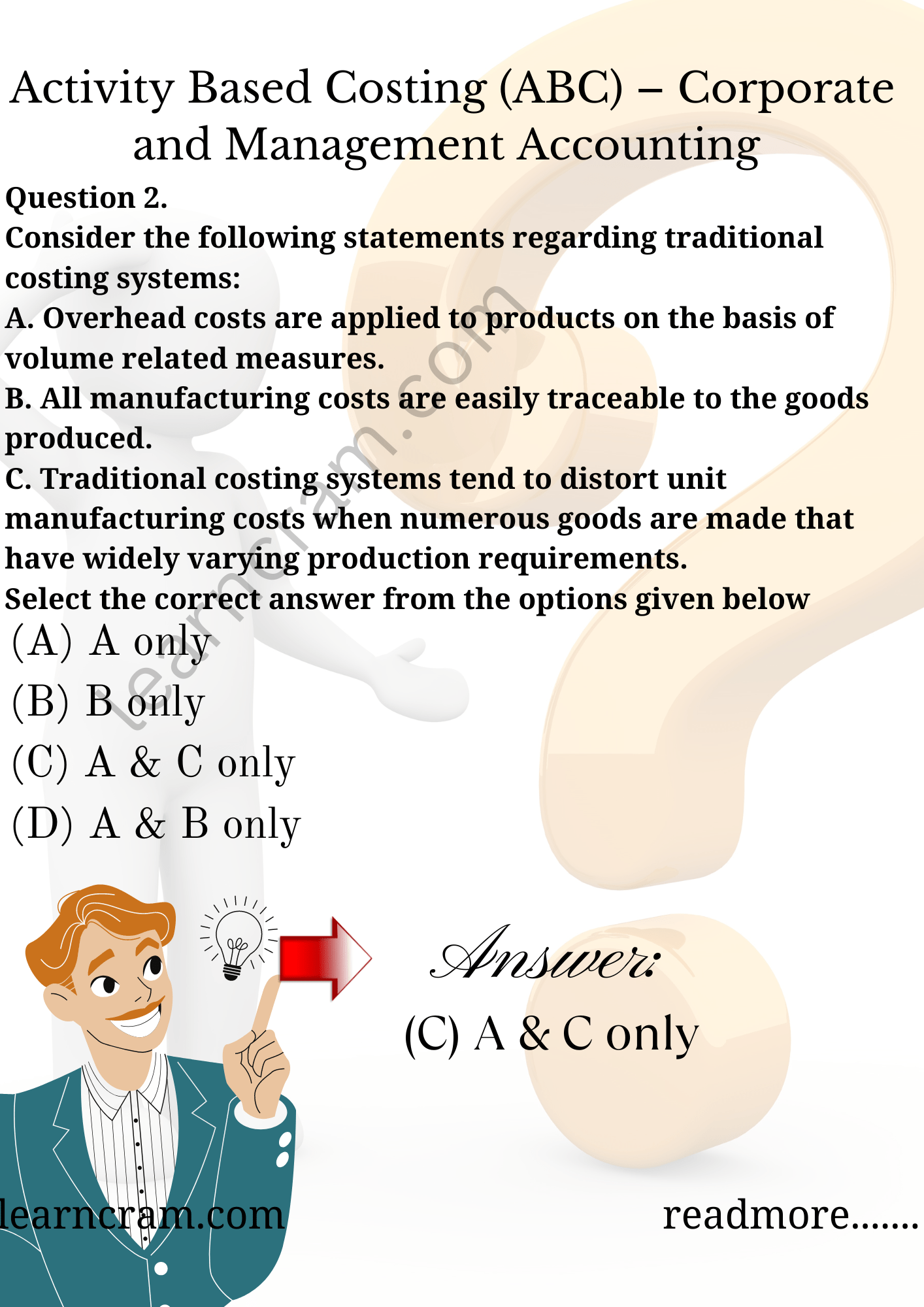Question 3.
The following tasks are associated with an activity-based costing system:
(1) Calculation of cost application rates
(2) Identification of cost drivers
(3) Assignment of cost to products
(4) Identification of cost pools
Which of the following choices correctly expresses the proper order of the preceding tasks?
(A) (1), (2), (3), (3)
(B) (4), (2), (1), (3)
(C) (4), (2), (3), (1)
(D) (2), (4), (1), (3)
(B) (4), (2), (1), (3)

Question 4.
…….. an item for which cost measurement is required e.g. product, job or a customer.
(A) Cost Pool
(B) Cost Driver
(C) Cost Absorption
(D) Cost Object
(D) Cost Object

Question 5.
In an ABC system, the allocation bases that are used for applying costs to services or procedures are called…………
(A) Cost Pool
(B) Cost Driver
(C) Cost Absorption
(D) Cost Object
(B) Cost Driver

Question 6.
Costs that are caused by a group of things being made, handled or processed at a single time are referred to as …….
(A) Unit Level Cost
(B) Batch Level Cost
(C) Product Level Cost
(D) Facility Level Cost
(B) Batch Level Cost

Question 7.
Cost of maintaining a building is
(A) Unit Level Cost
(B) Batch Level Cost
(C) Product Level Cost
(D) Facility Level Cost
(D) Facility Level Cost

Question 8
should be subtracted from net product revenues instead of an arbitrary and illogical apportionment.
(A) Facility Level Cost
(B) Product Level Cost
(C) Organizational Level Cost
(D) High Level Cost
(C) Organizational Level Cost

Question 9.
In traditional absorption costing system which of the following type activities are identified?
A. Unit Level Activities
B. Batch Level Activities
C. Product Level Activities
D. Facility Level Activities
Select the correct answer from the options given below.
(A) A & B
(B) B & C
(C) B & D
(D) A & D
(D) A & D

Question 10.
Which of the following tasks is not normally associated with an activity-based costing system?
(A) Calculation of cost application rates
(B) Identification of cost pools
(C) Preparation of allocation matrices
(D) Identification of cost drivers
(C) Preparation of allocation matrices

Question 11.
Which of the following is not a broad, cost classification category typically used in activity based costing?
(A) Facility Level
(B) Product Level
(C) Organizational Level
(D) Management Level
(D) Management Level

Question 12.
In an activity-based costing system, direct materials used would typically be classified as a:
(A) Unit Level Cost
(B) Batch Level Costs
(C) Product Level Cost
(D) Facility Level Cost
(A) Unit Level Cost

Question 13.
Which of the following is least likely to be classified as a batch level activity in an activity based costing system?
(A) Quality assurance
(B) Receiving and inspection
(C) Property taxes
(D) Production setup
(C) Property taxes

Question 14.
GODREJ, an appliance manufacturer, is developing a new line of ovens that uses controlled-laser technology. Research and testing costs associated with the new ovens is said to arise from a:
(A) Unit Level Activity
(B) Competitive Level Activity
(C) Facility Level Activity
(D) Product Sustaining Activity
(D) Product Sustaining Activity

Question 15.
Consider the following statements regarding product sustaining activities:
A.They must be done for each batch of product that is made.
B. They must be done for each unit of product that is made.
C. They are needed to support an entire product line.
Which of the above statements is/are true?
(A) A & B only
(B) B only
(C) C only
(D) A & C only
(C) C only

Question 16.
Which of the following is the proper sequence of events in an activity based costing system?
(A) Identification of cost drivers, identification of cost pools, calculation of cost application rates, assignment of cost to products.
(B) Identification of cost pools, identification of cost drivers, calculation of cost application rates, assignment of cost to products.
(C) Assignment of cost to products, identification of cost pools, identification of cost drivers, calculation of cost application rates.
(D) Calculation of cost application rates, identification of cost drivers, identification of cost pools, assignment of cost to products.
(B) Identification of cost pools, identification of cost drivers, calculation of cost application rates, assignment of cost to products.

Question 17.
The salaries of a manufacturing plant’s management are said to arise from:
(A) Unit Level Activities
(B) Batch Level Activities
(C) Product Sustaining Activities
(D) Facility Level Activities
(D) Facility Level Activities

Question 18.
The division of activities into unit level,batch level, product sustaining level, and facility level categories is commonly known as a ‘
(A) Cost Object
(B) Cost Application Method
(C) Cost Hierarch
(D) Cost Estimation Method
(C) Cost Hierarch

Question 19.
Basic types of cost pool allocations include:
(A) Allocation of costs to segments, products, and services
(B) Determining inputs for CVP models
(C) Establishing cash flows for capital budgeting analyses
(D) Reallocation of costs among service departments
(A) Allocation of costs to segments, products, and services

Question 20.
The main difference(s) between how traditional costing and activity based costing treat indirect manufacturing costs is/ are that
(A) Traditional costing uses only production volume based drivers while activity based costing uses only non production volume based drivers.
(B) Traditional costing treats only unit level costs as variable, while ABC systems treat unit level, batch level and product level costs as variable.
(C) Traditional cost allocations are usu-ally based on a plant wide overhead rate, while ABC systems use departmental overhead rates.
(D) (B) & (C)
(C) Traditional cost allocations are usually based on a plant wide overhead rate, while ABC systems use departmental overhead rates.

Question 21.
Activity based cost systems would probably provide the greatest benefits for organizations that use
(A) Job order costing
(B) Process costing
(C) Standard costing
(D) Historical costing
(A) Job order costing

Question 22.
Under a traditional costing system, which of the following costs would likely be classified as indirect with respect to the various products manufactured?
(A) Plant maintenance
(B) Factory supplies
(C) Machinery depreciation
(D) All of the above
(D) All of the above

Question 23.
PK Ltd. is changing from a traditional costing system to an activity based system. As a result of this action, which of the following costs would likely change from indirect to direct?
(A) Direct materials, factory supplies
(B) Production setup, finished-goods inspection & direct materials
(C) Production setup, finished-goods inspection and product shipping
(D) All of the above
(C) Production setup, finished-goods inspection and product shipping

Question 24.
Of the following organizations, activity based costing can be used by:
(A) Manufacturers
(B) Financial services firms
(C) Book publishers
(D) All of the above
(D) All of the above

Question 25.
Which of the following statements about activity based costing is false?
(A) Activity based costing cannot be used by service businesses.
(B) In comparison with traditional costing systems, activity based costing tends to use more cost pools and more cost drivers.
(C) In comparison with traditional costing systems, activity based costing results in less cost averaging of various diversified activities.
(D) In comparison with traditional-costing systems, activity based costing results in more costs being classified as direct costs.
(A) Activity based costing cannot be used by service businesses.

Question 26.
Review cost of commercial loan applications is cost.
(A) Unit level
(B) Facility level
(C) Batch level
(D) Product sustaining
(A) Unit level

Question 27.
All of the following are examples of product-level activities except:
(A) Human resource management
(C) Testing a prototype of a new product
(A) Human resource management

Question 28.
All of the following are examples of batch level activities except:
(A) Purchase order processing
(B) Setting up equipment
(C) The clerical activity associated with processing purchase orders to pro-duce an order for a standard product
(D) Worker recreational facilities
(D) Worker recreational facilities

Question 29.
Which of the following statement is/ are true?
(A) An activity based costing system is generally easier to implement and maintain than a traditional costing system.
(B) One of the goals of activity based management is the elimination of waste by allocating costs to products that waste resources.
(C) Activity based costing uses a number of activity cost pools, each of which is allocated to products on the basis of direct labour-hours.
(D) Activity rates in activity based costing are computed by dividing costs from the first stage allocations by the activity measure for each activity cost pool.
(D) Activity rates in activity based costing are computed by dividing costs from the first stage allocations by the activity measure for each activity cost pool.

Question 30.
Activity based costing systems:
(A) Use a single, volume-based cost driver.
(B) Assign overhead to products based on the products’ relative usage of direct labour.
(C) Often reveal products that were under or over cost by traditional costing systems.
(D) Typically use fewer cost drivers than more traditional costing systems.
(C) Often reveal products that were under or over cost by traditional costing systems

Question 31.
Which of the following activities is NOT a batch level activity?
(A) Processing purchase orders
(B) Designing products
(C) Receive raw materials from suppliers
(D) Setting up equipment
(B) Designing products

Question 32.
Which of the following characteristics would be an indicator that a company would benefit from switching to activity based costing?
(A) Only one homogenous product is produced on a continuous basis
(B) The existing cost system is reliable and have produced excellent results
(C) Overhead costs are high and increasing and no one seems to know why
(D) The costs of implementing ABC out-weigh the benefits
(C) Overhead costs are high and increasing and no one seems to know why

Question 33.
Which of the following is a limitation of activity-based costing?
(A) Costs are accumulated by each major activity
(B) A variety of activity measures are used
(C) All costs in an activity cost pool pertain to a single activity
(D) Activity based costing relies on the assumption that the cost in each cost pool is strictly proportional to its cost measure
(D) Activity based costing relies on the assumption that the cost in each cost pool is strictly proportional to its cost measure

Question 34.
Which costing system will trace the most costs as direct costs?
(A) Job Order Costing
(B) Process Costing
(C) Activity Based Costing
(D) Absorption Costing
(C) Activity Based Costing

Question 35.
Providing the power required to run production equipment is an example of a:
(A) Facility level activity
(B) Batch level activity
(C) Unit level activity
(D) Product level activity
(C) Unit level activity

Question 36.
All of the following are part of the activity based cost system hierarchy except for
(A) Input costs
(B) Batch level costs
(C) Product sustaining costs
(D) Facility sustaining cost
(A) Input costs

Question 37.
Activity based costinghas which benefit over traditional cost analysis for services firms
(A) Identifies high cost/low value activities
(B) Pinpoints differences in the costs of serving different customers
(C) Provides an overall assessment of average overhead costs
(D) Focuses on margins for the delivery of services by volume
(B) Pinpoints differences in the costs of serving different customers

Question 38.
Which one of the following statements is true about activity based costing and traditional costing system?
(A) In the activity based costing, as in traditional costing systems, non-manufacturing costs are not assigned to products.
(B) When there are batch level or product level costs, in comparison to a traditional cost system, an activity based costing system ordinarily will shift costs from high volume to low volume products.
(C) ABC is typically used as a replacement for a company’s traditional costing system.
(D) The first-stage allocation in activity-based costing is the process by which overhead costs are assigned to products before they are assigned to customers.
(B) When there are batch level or product level costs, in comparison to a traditional cost system, an activity based costing system ordinarily will shift costs from high volume to low volume products.

Question 39.
Which of the following is NOT a limitation of activity based costing?
(A) Maintaining an activity based costing system is more costly than maintaining a traditional direct labour based costing system.
(B) Changing from a traditional direct labour based costing system to an activity based costing system changes product margins and other key performance indicators used by managers. Such changes are often resisted by managers.
(C) In practice, most managers insist on fully allocating all costs to products, customers, and other costing objects in an activity based costing system. This results in overstated costs.
(D) More accurate product costs may result in increasing the selling prices of some products.
(D) More accurate product costs may result in increasing the selling prices of some products.

Question 40.
What does manufacturing overhead cost consist of?
(A) All manufacturing costs.
(B) All manufacturing costs, EXCEPT direct materials and direct labour.
(C) Indirect materials but NOT indirect labour.
(D) Indirect labour but NOT indirect materials.
(B) All manufacturing costs, EXCEPT direct materials and direct labour.

Question 41.
ZPALtd. customer service department follows up on customer complaints by telephone inquiry. During a recent period, the department initiated 7,000 calls and incurred costs of ₹ 2,03,000. If 2,940 of these calls were for the company’s wholesale operation (the remainder were for the retail division), costs allocated to the retail division should amount to:
(A) ₹ 85,260
(B) ₹ 1,17,740
(C) ₹ 2,03,000
(D) Nil
Use the following information to answer next 4 questions.
ABC Ltd. uses activity based costing to determine product costs for external financial reports. ABC Ltd. has provided the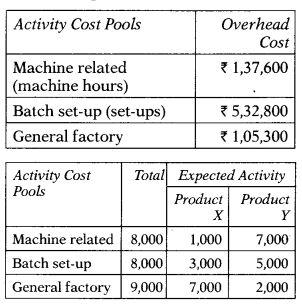(A) ₹ 85,260
Calls for retail division = 7,000 – 2,940 = 4,060 4,060
2,03,000 × $$\frac{4,060}{7,000}$$=1,17,740

Question 42.
The activity rate for the batch setup activity cost pool is closet to:
(A) ₹ 66.60
(B) ₹ 106.60
(C) ₹ 97.00
(D) ₹ 177.60
(A) ₹ 66.60
$$\frac{5,32,800}{8,000}$$=66.6

Question 43.
The activity rate for the Machine related activity cost pool is closet to:
(A) ₹ 66.60
(B) ₹ 106.60
(C) ₹ 97.00
(D) ₹ 17.20
(D) ₹ 17.20
$$\frac{1,37,600}{8,000}$$=17.2

Question 44.
The total amount of overhead cost allocated to Product X would be:
(A) ₹ 4,76,800
(B) ₹ 2,98,900
(C) ₹ 2,91,000
(D) ₹ 3,87,850
(B) ₹ 2,98,900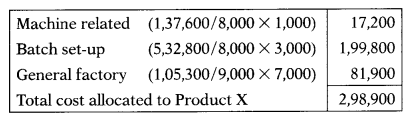Question 45.
The total amount of overhead cost allocated to Product Y would be:
(A) ₹ 2,98,900
(B) ₹ 5,33,000
(C) ₹ 4,76,800
(D) ₹ 2,91,000
(C) ₹ 4,76,800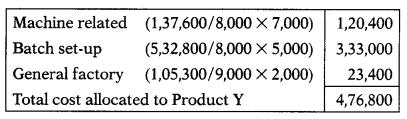Question 46.
A Company uses activity based costing to compute product costs for external reports. The company has three activity cost pools and applies overhead using predetermined overhead rates for each activity cost pool. Estimated costs and activities are presented below for the three activity cost pools:The amount of overhead for Activity 3 if current activity was 1,340:
(A) ₹ 38,519.00
(B) ₹ 39,704.20
(C) ₹ 38,564.00
(D) ₹ 23,876.80
Use the following information to answer next 2 questions.
Company Y estimated that it will incur a total overhead cost of 16,00,000. It considers implementing activity based costing. Three cost pools and respective activity measures have been identified: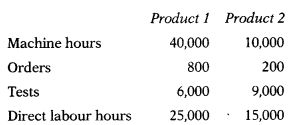The company currently uses traditional costing and allocates overhead based on direct labour hours.
(B)
38,519 × $$\frac{1,340}{1,300}$$=39,704

Question 47.
(A) 3,75000
(B) 3,00,000
(C) 3,25,000
(D) 2,25,000
(A) 3,75000
Total Overheads = 2,00,000 + 1,00,000 + 3,00,000 = 6,00,000
6,00,000 × $$\frac{1,200}{2,000}$$ =3,30,000

Question 48.
How much overhead would be assigned to Product 1 if activity based costing is used?
(A) ₹ 3,00,000
(B) ₹ 1,73,000
(C) ₹ 4,20,000
(D) ₹ 3,60,000
(D) ₹ 3,60,000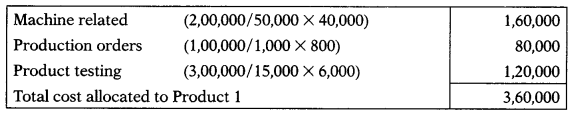Question 49.
Company X uses activity-based costing for its two products: Products B & D. One of the activity cost pools is parts administration. The total estimated overhead cost for that pool was ₹5,50,000 and the expected activity was 2,000 part types. If Product D requires 1,200 part types, the amount of overhead allocated to it would be:
(A) ₹ 2,75,000
(B) ₹ 3,00,000
(C) ₹ 3,30,000
(D) ₹ 3,45,000
(C) ₹ 3,30,000
5,50,000 ×$$\frac{1,200}{2,000}$$=3,30,000

Question 50.
One of company A’s cost pools is parts administration. The expected overhead cost for that cost pool was ₹ 3,80,000 and the expected activity was 5,000 part types. The actual overhead cost for the cost pool was ₹ 4,20,000 at an actual activity of 6,000 part types. The activity rate used to assign costs for that cost pool was:
(A) ₹ 63 per part type
(B) ₹ 76 per part type
(C) ₹ 70 per part type
(D) ₹ 84 per part type
Use the following information to answer next 9 questions.
Hi-Tech Products manufactures 3 types of DVD players: Economy, Standard & Deluxe. The company, which uses activity based costing, has identified 5 activities and related cost drivers. Each activity, its budgeted cost and related cost driver is identified below.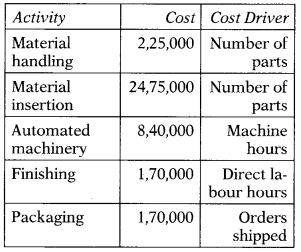The following information pertains to each product line of DVD players for next year(B)
$$\frac{3,80,000}{5,000}$$=76

Question 51.
What is Hi-Tech’s cost application rate for the material-handling activity?
(A) ₹ 1.00 per part
(B) ₹ 2.25 per part
(C) ₹ 2.25 per part
(D) ₹ 13.23 per part
(A) ₹ 1.00 per part
No. of parts = (10,000 × 10) + (5,000 × 15) + (2,000 × 25) = 2,25,000
Material Handing Activity Rate =$$\frac{2,25,000}{2,25,000}$$= ₹ 1 per part

Question 52.
What is Hi-Tech’s cost application rate for the automated machinery activity?
(A) ₹ 24.00 per machine hour
(B) ₹ 24.50 per labour hour
(C) ₹ 49.42 per unit
(D) ₹ 50.00 per machine hour
(A) ₹ 24.00 per machine hour
Machine Hours = (10,000 × 1) + (5,000 × 3) + (2,000 × 5) = 35,000
Machinery Activity Rate = $$\frac{8,40,000}{35,000}$$ = ₹24 per machine hour

Question 53.
What is Hi-Tech’s cost application rate for the finishing activity?
(A) ₹ 5.00 per labour hour
(B) ₹ 5.00 per machine hour
(C) ₹ 5.00 per unit
(D) ₹ 7.50 per unit
(A) ₹ 5.00 per labour hour
Labour Hours = (10,000 × 2) + (5,000 × 2) + (2,000 × 2) = 34,000
Finishing Activity Rate =$$\frac{1,70,000}{34,000}$$ = ₹ 5 per labour hour

Question 54.
What is Hi-Tech’s cost application rate for the packaging activity?
(A) ₹ 4.86 per machine hour
(B) ₹ 5.00 per labour hour
(C) ₹ 10.00 per unit
(D) ₹ 100.00 per order shipped
(D) ₹ 100.00 per order shipped
Packaging Activity Rate = $$\frac{1,70,000}{1,700}$$ = ₹ 100 per order shipped

Question 55.
Under an activity based costing system, what is the per unit cost of Economy?
(A) ₹ 141
(B) ₹ 164
(C) ₹ 225
(D) ₹ 228
(B) ₹ 164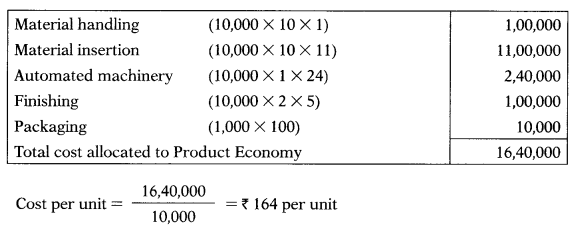Question 56.
Under an activity based costing system, what is the per unit cost of Standard?
(A) ₹ 164
(B) ₹ 228
(C) ₹ 272
(D) ₹282
(C) ₹ 272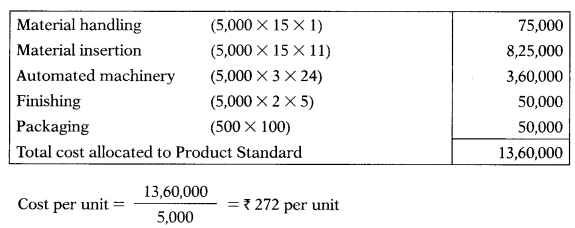Question 57.
Under an activity based costing system, what is the per-unit cost of Deluxe?
(A) ₹ 272
(B) ₹ 282
(C) ₹ 320
(D) ₹ 440
(D) ₹ 440

Question 58.
Assume that Hi-Tech is using a volume based costing system, and the preceding manufacturing costs are applied to all products based on direct labour hours. How much of the preceding cost is assigned to Deluxe?
(A) ₹ 4,56,471
(B) ₹ 6,46,471
(C) ₹ 9,61,176
(D) ₹ 11,41,176
(A) ₹ 4,56,471
38,80,000 × $$\frac{4,000}{34,000}$$ = 4,56,471

Question 59.
Assume that Hi-Tech is using a volume based costing system and the preceding manufacturing costs are applied to all products based on direct labour hours. How much of the preceding cost is assigned to Standard?
(A) ₹ 4,56,471
(B) ₹ 6,46,471
(C) ₹ 9,61,176
(D) ₹ 11,41,176
Use the following information to answer next 4 questions.
A company manufacturing two products A & B furnishes the following data for the year:The annual overheads are as under:
Volume related activity costs : ₹ 5,50,000
Set-up related costs :₹ 8,20,000
Purchase related costs : ₹ 6,18,000
(D)
38,80,000 ×$$\frac{10,000}{34,000}$$=11,41,176

Question 60.
Overhead cost per unit of Product A as per traditional absorption costing system = ?
(A) ₹ 28.40 per unit
(B) ₹ 24.52 per unit
(C) ₹ 56.80 per unit
(D) ₹ 103.32 per unit
(C) ₹ 56.80 per unit

Question 61.
Overhead cost per unit of Product B as per traditional absorption costing system = ?
(A) ₹ 28.40 per unit
(B) ₹ 24.52 per unit
(C) ₹ 56.80 per unit
(D) ₹ 103.32 per unit
(A) ₹ 28.40 per unit

Question 62.
Overhead cost per unit of Product A as per activity based costing system = ?
(A) ₹ 28.40 per unit
(B) ₹ 24.52 per unit
(C) ₹ 56.80 per unit
(D) ₹ 103.32 per unit
(D) ₹ 103.32 per unit

Question 63.
Overhead cost per unit of Product B as per activity based costing system = ?
(A) ₹ 24.40 per unit
(B) ₹ 24.52 per unit
(C) ₹ 24.80 per unit
(D) ₹ 23.32 per unit
(B) ₹ 24.52 per unit

Question 64.
JF Ltd. uses activity based costing to determine the costs of its two products: A & B. The estimated total cost and expected activity for one of the company’s three activity cost pools are as follows.The activity rate under the activity based costing system for this activity is closest to:
(A) ₹ 4.00
(B) ₹ 8.59
(C) ₹ 18.00
(D) ₹ 20.00
Use the following information to answer next 4 questions.
Best Chair Company makes two types of chairs, hand-built lounge chair (HC) and a folding beach chair (FC). The company had used a job order costing system and applies overhead on the basis of direct labour hours. Best Chair expects to produce 40,000 HC and 1,00,000 FC next year. Total direct material costs are ₹ 32,00,000 for HC and ₹ 10,00,000 FC. Best Chair has begun changing to an activity based costing system. The company has reported the following results from the first stage cost allocations for year’s production:
Labour related — 3,00,000
Machine related — 4,50,000
Machine set-up — 7,30,000
Order processing — 6,00,000
General factory — 5,00,000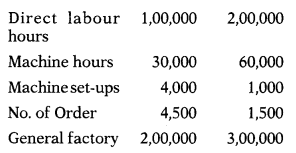(D)

Question 65.
What is direct labour rate per hour as per traditional costing system?
(A) ₹ 8.60 per direct labour hour
(B) ₹ 1.67 per direct labour hour
(C) ₹ 5.85 per direct labour hour
(D) ₹ 1.00 per direct labour hour
(A) ₹ 8.60 per direct labour hour

Question 66.
What is total unit cost per chair of HC as per traditional costing system?
(A) ₹ 37.10 per unit
(B) ₹ 167.10 per unit
(C) ₹ 157.20 per unit
(D) ₹ 101.50 per unit
(D) ₹ 101.50 per unit

Question 67.
What is total unit cost per chair of FC as per traditional costing system?
(A) ₹ 27.20 per unit
(B) ₹ 151.20 per unit
(C) ₹ 10.96 per unit
(D) ₹ 167.10 per unit
(A) ₹ 27.20 per unit

Question 68.
What is cost driver rate for labour related activity?
(A) ₹ 8.60 per direct labour hour
(B) ₹ 5.00 per direct labour hour
(C) ₹ 1.00 per direct labour hour
(D) ₹ 3.60 per direct labour hour
(C) ₹ 1.00 per direct labour hour

Question 69.
What is cost driver rate for order processing activity?
(A) ₹ 146.00 per order
(B) ₹ 100.00 per order
(C) ₹ 122.85 per order
(D) ₹ 144.67 per order
(A) ₹ 146.00 per order

Question 70.
What is total unit cost per chair of HC as per activity based costing system?
(A) ₹ 117.125 per unit
(B) ₹167.10 per unit
(C) ₹ 157.20 per unit
(D) ₹ 151.50 per unit
(A) ₹ 117.125 per unit

Question 71.
What is total unit cost per chair of FC as per activity based costing system?
(A) ₹ 57.20 per unit
(B) ₹ 151.20 per unit
(C) ₹ 20.96 per unit
(D) ₹ 167.10 per unit
(C) ₹ 20.96 per unit

Question 72.
Dec 2014: Cost attribution to cost units on the basis of benefit received from indirect activities, such as ordering, setting-up, assuring quality is known as:
(A) Allocation
(B) Activity based costing
(C) Always better control
(D) Absorption
(B) Activity based costing

Question 73.
Dec 2014: Which of the following is not included in batch level activities —
(A) Material ordering cost
(B) Machine set-up cost
(C) Inspection cost
(D) Designing the product
(D) Designing the product

Question 74.
Dec 2014: In activity based costing, the allocation basis used for applying costs to services or products is called —
(A) Cost driver
(B) Cost object
(C) Allocation
(D) Applicator
(A) Cost driver

Question 75.
June 2015: In activity based costing, an item for which cost measurement is required is called —
(A) Cost driver
(B) Cost object
(C) Allocation
(D) Cost pool
(B) Cost object

Question 76.
June 2015: Under activity based costing, ‘material ordering’ is considered as —
(A) Unit level activity
(B) Batch level activity
(C) Product level activity
(D) Facility level activity
(B) Batch level activity

Question 77.
June 2015: According to Chartered Institute of Management Accountants (CIMA), cost attribution to cost units on the basis of benefits received from indirect activities e.g. ordering, setting-up, assuring quality is known as —
(A) Absorption costing
(B) Marginal costing
(C) Activity based costing
(D) Job costing
(C) Activity based costing

Question 78.
Dec 2015: The basis of apportionment of overheads which takes into account the profitability of various departments is called —
(A) FIFO basis
(B) LIFO basis
(C) Ability to pay basis
(D) Activity basis
(D) Activity basis

Question 79.
Dec 2015: The key area(s) of activity based costing is/are —
(A) Product cost differentiation
(B) Identification of non-value added cost
(C) Distribution between fixed and variable cost
(D) Both (A) and (B) above
(D) Both (A) and (B) above

Question 80.
June 2016: Match the following events with type of activity:
Event —- Type of activity
(i) Material ordering — (A) Product level activity
(ii) Designing the product — (B) Facility level activity
(iii) Production manager salary — (C) Unit level activity
(iv) Use of consumables — (D) Batch level activity
Select the correct answer using the codes given below —(D)

Question 81.
Select the correct answer using the codes given below —
(i) Calculation of overheads application rates.
(ii) Identification of cost drivers
(iii) Identification of cost pools
(iv) Assignment of overheads cost to products.
Select the correct answer from the options given below —
(A) (i), (iii), (iv), (ii)
(B) (ii), (iii), (i), (iv)
(C) (iii), (ii), (i), (iv)
(D) (ii), (iii), (iv), (t)
(C) (iii), (ii), (i), (iv)

Question 82.
Dec 2016: Which of the following is the main cost driver of customer order processing activity —
(A) Flow of the product from assembly line
(B) Order value
(C) Number of problem suppliers
(D) Number of machine charges
(B) Order value

Question 83.
Dec 2016:
Assertion (A)
Activity based costing is not normally used for external reporting purpose.
Reason (R)
Activity based costing does not conform to generally accepted principles.
Select the correct answer from the options given below —
(A) Both A and R are true and R is the correct explanation of A
(B) Both A and R are true but R is not the correct explanation of A
(C) A is true, but R is false
(D) A is false, but R is true
(A) Both A and R are true and R is the correct explanation of A

Question 84.
Dec 2016: A homogeneous cost pool is one that —
(A) Does not change over time
(B) Needs many activity drivers to be allocated to a cost object
(C) Can be explained with a single activity driver
(D) Has only one type of material assigned to it
(C) Can be explained with a single activity driver

Question 85.
Dec 2017: In Traditional absorption costing system cost are first traced to:
(A) Activities
(B) Organizational unit
(C) Products
(D) Cost centres
(B) Organizational unit

Question 86.
Dec 2017: The main reason for the usage of Activity Based Costing, by replacing the traditional costing system is that:
(A) The overhead recovery rates used in traditional costing system are inappropriate for decision-making
(B) The companies deal with more number of product at present
(C) No scope for cause and effect relationship in traditional costing
(D) The new manufacturing technology needs information for feedback of performance even the product is in progress.
(B) The companies deal with more number of product at present

Question 87.
Dec 2017: In Activity Based Costing, inspection of product is a ……… level activity.
(A) Unit
(B) Batch
(C) Product
(D) Facility
(B) Batch

Question 88.
Dec 2017:
Assertion (A):
Implementation of ABC System requires substantial resources which are costly to maintain.
Reason (R):
Activity Based Costing is a two-stage product costing.
Select the correct answer from the options given below:
(A) Both A and R are true and R is the correct explanation of A
(B) Both A and R are true but R is not the correct explanation of A
(C) A is true, but R is false
(D) A is false, but R is true
(B) Both A and R are true but R is not the correct explanation of A

Question 89.
Dec 2017: Match the following:
List – I — List – II
(a) Unit level activity (1) Material ordering
(b) Batch level activity (2) Plant security
(c) Product level activity (3) Use of indirect material
(d) Facility level activity (4) Parts management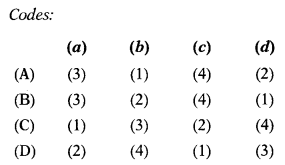(A)

Question 90.
Dec 2017: Fast Ltd., manufactures three types of products A, B and C following ABC System. During a period the company incurred ₹ 73,000 as inspection cost and it was worked for 10, 20 and 9 production runs respectively for producing products A, B and C. The inspection costs for product B under ABC system was:
(A) ₹ 15,000
(B) ₹ 40,000
(C) ₹ 18,000
(D) ₹ 24,000
(B) ₹ 40,000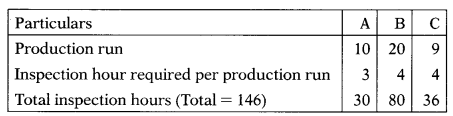Inspection cost per hour = 73,000 = 146 = 500.
Inspection cost for Product B = 20 × 4 × 500 = 40,000.

Question 91.
June 2018: Which of the following statement is not true in respect of Activity based costing?
(A) Activity based costing improves control over overheads costs.
(B) Setting up equipment is a batch level activity.
(C) In Activity based costing each cost pool has its own predetermined overhead rate.
(D) Activity based costing is less expensive to implement than traditional costing.
(D) Activity based costing is less expensive to implement than traditional costing.

Question 92.
June 2018: Performing periodic maintenance on buildings and general use equipments is an example of:
(A) Facility level activity
(B) Unit level activity
(C) Batch level activity
(D) Product level activity
(B) Unit level activity

Question 93.
June 2018: The division of activities into unit level, batch level, product level, and facility level categories is commonly known as a:
(A) Cost object
(B) Cost application
(C) Cost hierarchy
(D) Cost estimation
(B) Cost application

Question 94.
Dec 2018: Costs which are caused by a group of things being made or processed at a single time are referred to as:
(A) Product-level costs
(B) Cost pool
(C) Organizational-level costs
(D) Batch level costs
(D) Batch level costs

Question 95.
Dec 2018: Level Activities are identified in traditional Absorption Costing.
(A) Unit
(B) Batch
(C) Product
(A) Unit

Question 96.
Dec 2018: encourages managers to identify which activities are value added activities.
(A) Standard Costing
(B) Activity-based Costing
(C) Uniform Costing
(D) Direct Costing
(B) Activity-based Costing

Question 97.
Dec 2018: ABC emphasize on:
(A) More precise profit analysis
(B) More accurate costing
(C) Improved cost control
(D) All of the above three